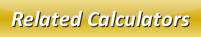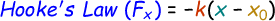# Hooke's Law Calculator

The hooke's law is the principle which explains the stress applied on any solid material is directly proportional to the generated strain within the limit of elasticity.

The hooke's law formula is given below:where,
Fx = Force [N]
k = Spring Force Constant [n/m]
x = Distance from Equilibrium [m]
x0 = Spring Equilibrium Position [m]

In our below online hooke's law calculator, enter the given input values and click calculate button to find the force.

 Spring Force Constant (k): [N/m] Distance from Equilibrium (x): [m] Spring Equilibrium Position (x0): [m] Force (Fx): [N]

Latest Calculator Release

Average Acceleration Calculator

Average acceleration is the object's change in speed for a specific given time period. ...

Free Fall Calculator

When an object falls into the ground due to planet's own gravitational force is known a...

Torque Calculator

Torque is nothing but a rotational force. In other words, the amount of force applied t...

Average Force Calculator

Average force can be explained as the amount of force exerted by the body moving at giv...

Angular Displacement Calculator

Angular displacement is the angle at which an object moves on a circular path. It is de...